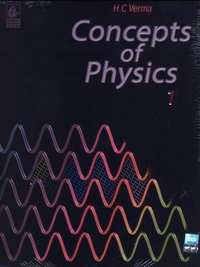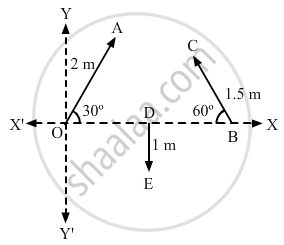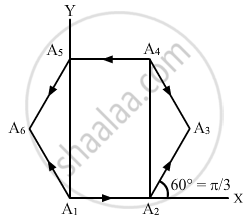CBSE (Science) Class 11CBSE
Share

# H.C. Verma solutions for Class 12 Physics chapter 2 - Physics and Mathematics

## Concept of Physics Part-1 (2018-2019 Session) by H.C Verma

#### H.C. Verma Concept of Physics Part-1 (2018-2019 Session) by H.C Verma## Chapter 2: Physics and Mathematics

#### Chapter 2: Physics and Mathematics Exercise Short Answers solutions [Pages 27 - 28]

Short Answers | Q 1 | Page 27

Is a vector necessarily changed if it is rotated through an angle ?

Short Answers | Q 2 | Page 27

Is it possible to add two vectors of unequal magnitudes and get zero? Is it possible to add three vectors of equal magnitudes and get zero?

Short Answers | Q 3 | Page 27

Does the phrase "direction of zero vector" have physical significance? Discuss it terms of velocity, force etc.

Short Answers | Q 4 | Page 27

Can you add three unit vectors to get a unit vector? Does your answer change if two unit vectors are along the coordinate axes?

Short Answers | Q 5 | Page 28

Can we have physical quantities having magnitude and direction which are not vectors?

Short Answers | Q 6 | Page 28

Which of the following two statements is more appropriate?
(a) Two forces are added using triangle rule because force is a vector quantity.
(b) Force is a vector quantity because two forces are added using triangle rule.

Short Answers | Q 7 | Page 28

Can you add two vectors representing physical quantities having different dimensions? Can you multiply two vectors representing physical quantities having different dimensions?

Short Answers | Q 8 | Page 28

Can a vector have zero component along a line and still have nonzero magnitude?

Short Answers | Q 9 | Page 28

Let ε1 and ε2 be the angles made by  $\vec{A}$ and -$\vec{A}$ with the positive X-axis. Show that tan ε1 = tan ε2. Thus, giving tan ε does not uniquely determine the direction of $\vec{A}$.

Short Answers | Q 10 | Page 28

Is the vector sum of the unit vectors  $\vec{i}$ and $\vec{i}$ a unit vector? If no, can you multiply this sum by a scalar number to get a unit vector?

Short Answers | Q 11 | Page 28

Let $\vec{A} = 3 \vec{i} + 4 \vec{j}$. Write a vector $\vec{B}$ such that $\vec{A} \neq \vec{B}$, but A = B.

Short Answers | Q 12 | Page 28

Can you have  $\vec{A} \times \vec{B} = \vec{A} \cdot \vec{B}$ with A ≠ 0 and B ≠ 0 ? What if one of the two vectors is zero?

Short Answers | Q 13 | Page 28

If $\vec{A} \times \vec{B} = 0$ can you say that

(a) $\vec{A} = \vec{B} ,$

(b) $\vec{A} \neq \vec{B}$ ?

Short Answers | Q 14 | Page 28

Let $\vec{A} = 5 \vec{i} - 4 \vec{j} \text { and } \vec{B} = - 7 \cdot 5 \vec{i} + 6 \vec{j}$. Do we have $\vec{B} = k \vec{A}$ ? Can we say $\frac{\vec{B}}{\vec{A}}$ = k ?

#### Chapter 2: Physics and Mathematics Exercise MCQ solutions [Page 28]

MCQ | Q 1 | Page 28

A vector is not changed if

• it is rotated through an arbitrary angle

• it is multiplied by an arbitrary scalar

•  it is cross multiplied by a unit vector

• it is slid parallel to itself.

MCQ | Q 2 | Page 28

Which of the sets given below may represent the magnitudes of three vectors adding to zero?

• 2, 4, 8

• 4, 8, 16

•  1, 2, 1

• 0.5, 1, 2

MCQ | Q 3 | Page 28

The resultant of  $\vec{A} \text { and } \vec{B}$ makes an angle α with  $\vec{A}$ and β with $\vec{B}$,

• α < β

• α < β if A < B

•  α < β if A > B

• α < β if A = B

MCQ | Q 4 | Page 28

The component of a vector is

• always less than its magnitude

• always greater than its magnitude

• always equal to its magnitude

• None of these.

MCQ | Q 5 | Page 28

A vector $\vec{A}$ points vertically upward and $\vec{B}$ points towards the north. The vector product $\vec{A} \times \vec{B}$ is

• along the west

• along the east

• zero

• vertically downward.

MCQ | Q 6 | Page 28

The radius of a circle is stated as 2.12 cm. Its area should be written as

• 14 cm2

• 14.1 cm2

• 14.11 cm2

• 14.1124 cm2

#### Chapter 2: Physics and Mathematics Exercise MCQ solutions [Page 28]

MCQ | Q 1 | Page 28

A situation may be described by using different sets coordinate axes having different orientation. Which the following do not depended on the orientation of the axis?
(a) the value of a scalar
(b) component of a vector
(c) a vector
(d) the magnitude of a vector.

MCQ | Q 2 | Page 28

Let $\vec{C} = \vec{A} + \vec{B}$

• $\left| \vec{C} \right|$ is always greater than $\left| \vec{A} \right|$

• It is possible to have $\left| \vec{C} \right|$  < $\left| \vec{A} \right|$ and $\left| \vec{C} \right|$ < $\left| \vec{B} \right|$

• C is always equal to A + B

• C is never equal to A + B.

MCQ | Q 3 | Page 28

Let the angle between two nonzero vectors $\vec{A}$ and $\vec{B}$ be 120° and its resultant be $\vec{C}$.

• C must be equal to $\left| A - B \right|$

•  C must be less than $\left| A - B \right|$

• C must be greater than $\left| A - B \right|$

• C may be equal to $\left| A - B \right|$

MCQ | Q 4 | Page 28

The x-component of the resultant of several vectors
(a) is equal to the sum of the x-components of the vectors of the vectors
(b) may be smaller than the sum of the magnitudes of the vectors
(c) may be greater than the sum of the magnitudes of the vectors
(d) may be equal to the sum of the magnitudes of the vectors.

MCQ | Q 5 | Page 28

The magnitude of the vector product of two vectors $\left| \vec{A} \right|$ and $\left| \vec{B} \right|$ may be

(a) greater than AB
(b) equal to AB
(c) less than AB
(d) equal to zero.

#### Chapter 2: Physics and Mathematics Exercise Exercise solutions [Pages 29 - 30]

Exercise | Q 1 | Page 29

A vector $\vec{A}$ makes an angle of 20° and $\vec{B}$ makes an angle of 110° with the X-axis. The magnitudes of these vectors are 3 m and 4 m respectively. Find the resultant.

Exercise | Q 2 | Page 29

Let $\vec{A} \text { and } \vec{B}$ be the two vectors of magnitude 10 unit each. If they are inclined to the X-axis at angle 30° and 60° respectively, find the resultant.

Exercise | Q 3 | Page 29

Add vectors $\vec{A} , \vec{B} \text { and } \vec{C}$  each having magnitude of 100 unit and inclined to the X-axis at angles 45°, 135° and 315° respectively.

Exercise | Q 4 | Page 29

Let $\vec{a} = 4 \vec{i} + 3 \vec{j} \text { and } \vec{b} = 3 \vec{i} + 4 \vec{j}$. Find the magnitudes of (a)  $\vec{a}$ ,  (b)  $\vec{b}$ ,(c) $\vec{a} + \vec{b} \text { and }$ (d) $\vec{a} - \vec{b}$.

Exercise | Q 5 | Page 29

Refer to figure (2-E1). Find (a) the magnitude, (b) x and y component and (c) the angle with the X-axis of the resultant of $\overrightarrow{OA} , \overrightarrow{BC} \text { and } \overrightarrow{DE}$.Exercise | Q 6 | Page 29

Two vectors have magnitudes 2 unit and 4 unit respectively. What should be the angle between them if the magnitude of the resultant is (a) 1 unit, (b) 5 unit and (c) 7 unit.

Exercise | Q 7 | Page 29

A spy report about a suspected car reads as follows. "The car moved 2.00 km towards east, made a perpendicular left turn, ran for 500 m, made a perpendicular right turn, ran for 4.00 km and stopped". Find the displacement of the car.

Exercise | Q 8 | Page 29

A carrom board (4 ft × 4 ft square) has the queen at the centre. The queen, hit by the striker moves to the from edge, rebounds and goes in the hole behind the striking line. Find the magnitude of displacement of the queen (a) from the centre to the front edge, (b) from the front edge to the hole and (c) from the centre to the hole.

Exercise | Q 9 | Page 29

A mosquito net over a 7 ft × 4 ft bed is 3 ft high. The net has a hole at one corner of the bed through which a mosquito enters the net. It flies and sits at the diagonally opposite upper corner of the net. (a) Find the magnitude of the displacement of the mosquito. (b) Taking the hole as the origin, the length of the bed as the X-axis, it width as the Y axis, and vertically up as the Z-axis, write the components of the displacement vector.

Exercise | Q 10 | Page 29

Suppose $\vec{a}$ is a vector of magnitude 4.5 units due north. What is the vector (a) $3 \vec{a}$, (b) $- 4 \vec{a}$ ?

Exercise | Q 11 | Page 29

Two vectors have magnitudes 2 m and 3m. The angle between them is 60°. Find (a) the scalar product of the two vectors, (b) the magnitude of their vector product.

Exercise | Q 12 | Page 29

Let A1 A2 A3 A4 A5 A6 A1 be a regular hexagon. Write the x-components of the vectors represented by the six sides taken in order. Use the fact the resultant of these six vectors is zero, to prove that
cos 0 + cos π/3 + cos 2π/3 + cos 3π/3 + cos 4π/3 + cos 5π/3 = 0.
Use the known cosine values to verify the result.Exercise | Q 13 | Page 29

Let $\vec{a} = 2 \vec{i} + 3 \vec{j} + 4 \vec{k} \text { and } \vec{b} = 3 \vec{i} + 4 \vec{j} + 5 \vec{k}$ Find the angle between them.

Exercise | Q 14 | Page 29

Prove that $\vec{A} . \left( \vec{A} \times \vec{B} \right) = 0$.

Exercise | Q 15 | Page 29

If  $\vec{A} = 2 \vec{i} + 3 \vec{j} + 4 \vec{k} \text { and } \vec{B} = 4 \vec{i} + 3 \vec{j} + 2 \vec{k}$ find $\vec{A} \times \vec{B}$.

Exercise | Q 16 | Page 29

If $\vec{A} , \vec{B} , \vec{C}$ are mutually perpendicular, show that  $\vec{C} \times \left( \vec{A} \times \vec{B} \right) = 0$ Is the converse true?

Exercise | Q 17 | Page 29

A particle moves on a given straight line with a constant speed ν. At a certain time it is at a point P on its straight line path. O is a fixed point. Show that $\vec{OP} \times \vec{\nu}$ is independent of the position P.

Exercise | Q 18 | Page 29

The force on a charged particle due to electric and magnetic fields is given by $\vec{F} = q \vec{E} + q \vec{\nu} \times \vec{B}$.
Suppose $\vec{E}$  is along the X-axis and $\vec{B}$  along the Y-axis. In what direction and with what minimum speed ν should a positively charged particle be sent so that the net force on it is zero?

Exercise | Q 19 | Page 29

Give an example for which $\vec{A} \cdot \vec{B} = \vec{C} \cdot \vec{B} \text{ but } \vec{A} \neq \vec{C}$.

Exercise | Q 20 | Page 29

Draw a graph from the following data. Draw tangents at x = 2, 4, 6 and 8. Find the slopes of these tangents. Verify that the curve draw is y = 2x2 and the slope of tangent is $\tan \theta = \frac{dy}{dx} = 4x$
$\begin{array}x & 1 & 2 & 3 & 4 & 5 & 6 & 7 & 8 & 9 & 10 \\ y & 2 & 8 & 18 & 32 & 50 & 72 & 98 & 128 & 162 & 200\end{array}$

Exercise | Q 21 | Page 29

A curve is represented by y = sin x. If x is changed from $\frac{\pi}{3}\text{ to }\frac{\pi}{3} + \frac{\pi}{100}$ , find approximately the change in y.

Exercise | Q 22 | Page 29

The electric current in a charging R−C circuit is given by i = i0 e−t/RC where i0, R and C are constant parameters of the circuit and t is time. Find the rate of change of current at (a) t = 0, (b) t = RC, (c) t = 10 RC.

Exercise | Q 23 | Page 29

The electric current in a discharging R−C circuit is given by i = i0 e−t/RC where i0, R and C are constant parameters and t is time. Let i0 = 2⋅00 A, R = 6⋅00 × 105 Ω and C = 0⋅500 μF. (a) Find the current at t = 0⋅3 s. (b) Find the rate of change of current at at 0⋅3 s. (c) Find approximately the current at t = 0⋅31 s.

Exercise | Q 24 | Page 29

Find the area bounded under the curve y = 3x2 + 6x + 7 and the X-axis with the ordinates at x = 5 and x = 10.

Exercise | Q 25 | Page 30

Find the area enclosed by the curve y = sin x and the X-axis between x = 0 and x = π.

Exercise | Q 26 | Page 30

Find the area bounded by the curve y = ex, the X-axis and the Y-axis.

Exercise | Q 27 | Page 29

A rod of length L is placed along the X-axis between x = 0 and x = L. The linear density (mass/length) ρ of the rod varies with the distance x from the origin as ρ = a + bx. (a) Find the SI units of a and b. (b) Find the mass of the rod in terms of a, b and L.

Exercise | Q 28 | Page 30

The momentum p of a particle changes with time t according to the relation
$\frac{dp}{dt} = \left( 10 N \right) + \left( 2 N/s \right)t$ If the momentum is zero at t = 0, what will the momentum be at t = 10 s?

Exercise | Q 29 | Page 30

The changes in a function y and the independent variable x are related as
$\frac{dy}{dx} = x^2$ . Find y as a function of x.

Exercise | Q 30 | Page 30

Write the number of significant digits in (a) 1001, (b) 100.1, (c) 100.10, (d) 0.001001.

Exercise | Q 31 | Page 30

A metre scale is graduated at every millimetre. How many significant digits will be there in a length measurement with this scale?

Exercise | Q 32 | Page 30

Round the following numbers to 2 significant digits.
(a) 3472, (b) 84.16. (c)2.55 and (d) 28.5

Exercise | Q 33 | Page 30

The length and the radius of a cylinder measured with a slide callipers are found to be 4.54 cm and 1.75 cm respectively. Calculate the volume of the cylinder.

Exercise | Q 34 | Page 30

The thickness of a glass plate is measured to be 2.17 mm, 2.17 mm and 2.18 mm at three different places. Find the average thickness of the plate from this data.

Exercise | Q 35 | Page 30

The length of the string of a simple pendulum is measured with a metre scale to be 90.0 cm. The radius of the bod plus the length of the hook is calculated to be 2.13 cm using measurements with a slide callipers. What is the effective length of the pendulum? (The effective length is defined as the distance between the point of suspension and the centre of the bob.)

## Chapter 2: Physics and Mathematics

#### H.C. Verma Concept of Physics Part-1 (2018-2019 Session) by H.C Verma## H.C. Verma solutions for Class 12 Physics chapter 2 - Physics and Mathematics

H.C. Verma solutions for Class 12 Physics chapter 2 (Physics and Mathematics) include all questions with solution and detail explanation. This will clear students doubts about any question and improve application skills while preparing for board exams. The detailed, step-by-step solutions will help you understand the concepts better and clear your confusions, if any. Shaalaa.com has the CBSE Concept of Physics Part-1 (2018-2019 Session) by H.C Verma solutions in a manner that help students grasp basic concepts better and faster.

Further, we at Shaalaa.com provide such solutions so that students can prepare for written exams. H.C. Verma textbook solutions can be a core help for self-study and acts as a perfect self-help guidance for students.

Concepts covered in Class 12 Physics chapter 2 Physics and Mathematics are Fundamental Forces in Nature, Physics, Technology and Society, Concept of Physics, Scope and Excitement of Physics, Nature of Physical Laws.

Using H.C. Verma Class 12 solutions Physics and Mathematics exercise by students are an easy way to prepare for the exams, as they involve solutions arranged chapter-wise also page wise. The questions involved in H.C. Verma Solutions are important questions that can be asked in the final exam. Maximum students of CBSE Class 12 prefer H.C. Verma Textbook Solutions to score more in exam.

Get the free view of chapter 2 Physics and Mathematics Class 12 extra questions for Physics and can use Shaalaa.com to keep it handy for your exam preparation

S